# Trig Identities : Table of Trigonometric Identities

Students are taught about trig identities or trigonometric identities in school and are an important part of higher-level mathematics. So to help you understand and learn all trig identities we have explained here all the concepts of trigonometry. As a student, you would find the trig identity sheet we have provided here useful. So you can download and print the identities PDF and use it anytime to solve the equations.
Trigonometry is an important branch of mathematics that deals with relationships between the lengths and angles of triangles. It is quite an old concept and was first used in the 3rd century BC. This branch of mathematics is related to planar right-triangles (or the right-triangles in a two-dimensional plane with one angle equal to 90 degrees).
There are some other branches where trigonometry has contributed immensely in its growth and development. Some of its fields of application are ;
• In music: It can be used to develop music digitally, through computer music.
• In aviation: it is of vital importance to lead an aircraft in the right direction. For instance, if the wind speed and the angle of the aircraft are known, it can be used to determine the direction of the aircraft.
• In criminology – trigonometry can also be used in criminology where it is used to calculate various important determinants of a crime scene, such as the trajectory of a projectile, how an object falls, etc.
• Mathematics: Trigonometry is one of the most important branches of mathematics, without which some other vital branches couldn’t have existed. For example, calculus is purely based upon trigonometry and algebra.

## What Are Trig Identities?

Although trigonometry does not have any direct application its application in our daily lives cannot be neglected. It is an indispensable aspect of many areas of studies and industries. Its most common application is to measure the height of a building, mountain or a tall object at a distance. The only two information required to find out the height is the angle of elevation and distance from the object.Trigonometric identities are mathematical equations which are made up of functions. These identities are true for any value of the variable put.
There are many identities which are derived by the basic functions, i.e., sin, cos, tan, etc. The most basic identity is the Pythagorean Identity, which is derived from the Pythagoras Theorem. It is used to determine the equations by applying the Pythagoras Theorem. So it helps us to determine the relationship between lines and angles in a right-angled triangle.
The other important identities are Hyperbolic identities, half-angle identities, inverse identities, etc.

## Hyperbolic Trig IdentitiesPDF

A hyperbolic function is similar to a function but might differ to it in certain terms. The basic hyperbolic functions are hyperbola sin and hyperbola cosine from which the other functions are derived.

So here we have provided a Hyperbola graph thus giving you an idea about the positions of sine, cosine, etc.

### Pythagorean Trig IdentitiesPythagoras Identities are the identities representing the Pythagoras Theorem in the form of functions. So these identities help us to basically determine the relationship between various sine and cosine functions. From there, you can derive the function of other identities as well.
The fundamental Pythagorean Trigonometric identity is :
sin(x) + cos(x) = 1
So from this formula, we can derive the formulas for other functions also :## Inverse Trig Identities

The inverse trigonometric functions are also called the arcus functions. Basically, they are the trig reciprocal identities of sin, cos, tan and other functions. These identities are used in situations when the domain of the function needs to be restricted.

These identities have special significance in engineering, navigation, physics, and geometry.

Here we have provided you with the table showing the Inverse identities of all the trigonometric functions :PDF

### Basic Trig Identities

The basic identities are the equations involving functions that are true every time for variables. So these identities depict certain functions of one or more angles. It is related to a unit circle where the relationship between the lines and angles in a Cartesian plane is depicted. Here we have provided you with the table consisting of a set of identities which can be derived from the basic functionsPDF

### Trig Double Identities

The double identities deal with the double angles of the identities. For example, sin(2A), cos (2A), tan(2A), etc. This is a special case where the sum of angles is obtained to get a double angle.

Here we have provided you with a chart showing all the double-angle formulas of functions :

##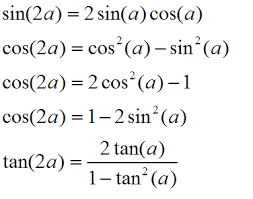PDF

### Trig Integral Identities

Integral Identities are the anti-derivative functions of their identities. If we apply the rules of differentiation to the basic functions, we get the integrals of the functions.

Here is a table depicting the indefinite integrals of various equations :

##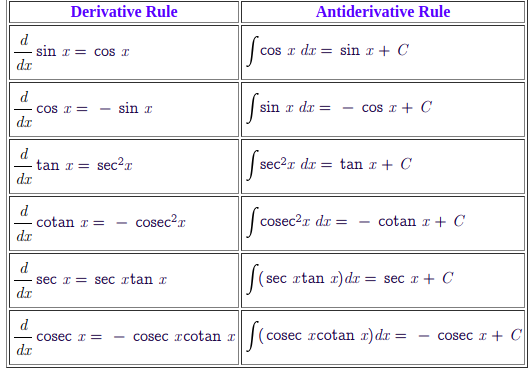PDF

### Trig Identities And Derivatives

Derivatives in Mathematics is the process of showing the rate of change of a function with respect to a variable at one given point of time. So derivatives imply the process of finding the derivatives of the functions.

Here we shall provide you with the list of derivatives of all the functions :PDF

### List of Trig Identities

Trigonometry is a wide branch that has applications in various field such as Mathematics, Physics, Astronomy, etc. Hence many identities or equalities have been derived by the mathematicians over the years from the basic functions. Here we shall provide you with the trig formulas list which contains all the identities used in Mathematics. So this trigonometry formula sheet will help you solve the complex equations.

##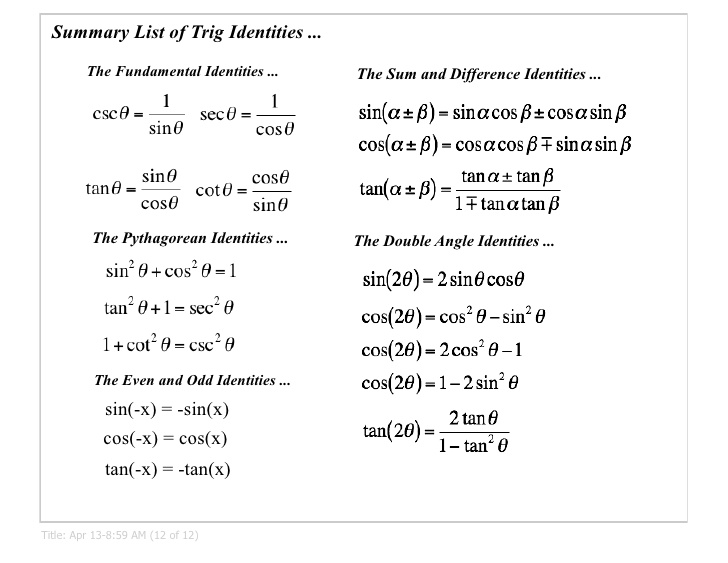PDF

### Trig Half-Angle Identities

The half-angle identities are the identities involving functions with half angles. The square root of the first two functions sine and cosine take negative or positive value depending upon the quadrant in which θ/2 lies.

Here is a table depicting the half-angle identities of all functions.

##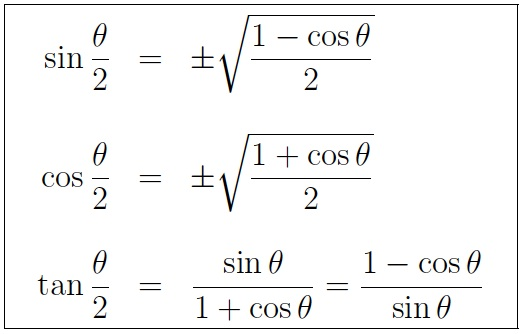PDF

### Fundamental Trig Identities

Fundamental identities comprise of various identities which are useful in solving complex problems. These basic identities are used to establish different relations between the functions.

Here is a chart showing the different fundamental identities.

PDF

## Trig Identities Chart

A chart form is very helpful for students to learn all the identities. A identities chart is useful since it shows the common trig identities in one place.

Here we are providing you with an identities chart which has all the formulas for identities given neatly.

PDF

## Trig Identities Worksheet

Now that you have learned about all the identities involving the formulas, you can use them, to solve the problems. Students will find it useful to recollect their concepts and assess their knowledge in trigonometry.

Here are identities worksheet which you can solve to understand the derivation of the identities.

PDF

## How To Verify Trig Identities

Verifying any formula is a difficult task since one formula leads to the derivation of others. So to verify trig identities, it is like any other equation and you have to deduce the identities logically from the other theorems.

Here through this video, we have explained to you how to prove trig identities.

## Trig Identities Table

There are many identities which are used to establish different relations among the functions. Learning all the identities can be tough for the students. So here we have provided you with the identities table which covers all the identities of Trig

PDF

### Double and Triple-Angle FormulasPDF

### Sum and Difference of AnglesPDF

### Trig Identities Cheat Sheet

A cheat sheet is very useful for students or any learner if they want to learn all the concepts of a topic in a short period of time. So a cheat sheet can be made for all the identities too. It will save the time and effort of students in understanding the concepts and help them perform better in exams.

Given below here is identities cheat sheet which has all information about functions and formulas written in brief. SO you can download it and carry it with you anywhere.

##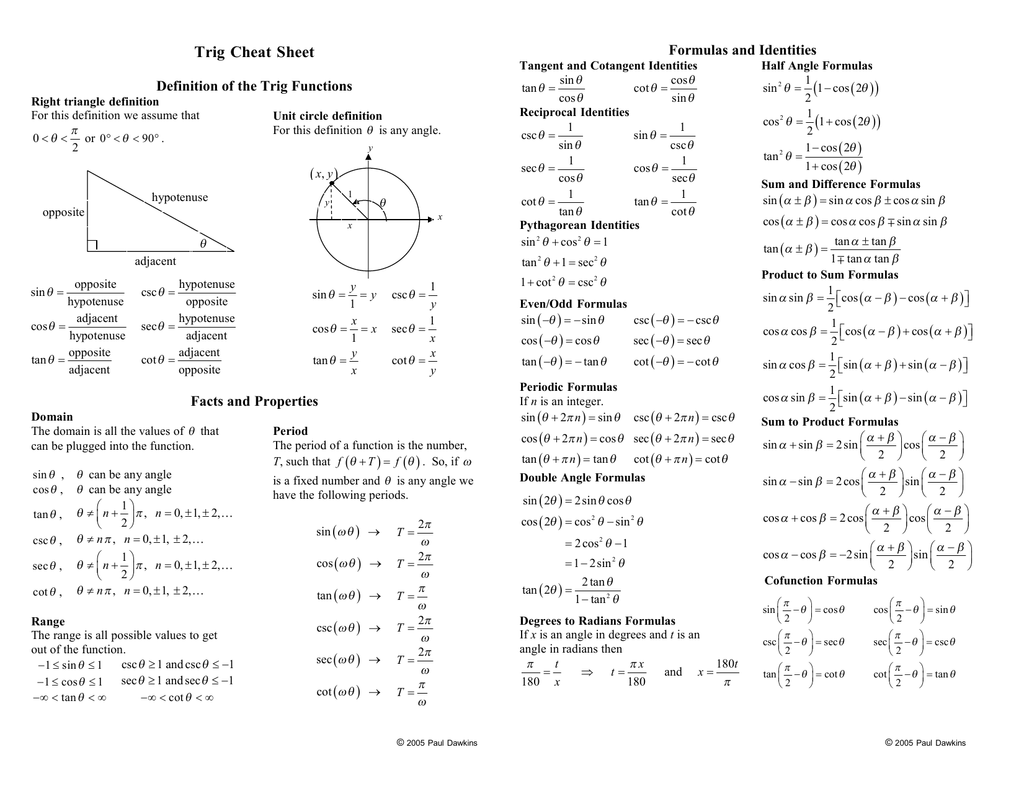PDF

### How To Use Trig Identities Calculator – Trigonometric Identities Solver

Sometimes while solving equations our L.H.S. might not match with the R.H.S. To perform such complicated calculations, an ordinary calculator is not sufficient and Identities Calculator is most suitable for the purpose.

A trigonometric calculator has the options of performing all the complex functions such as log, inverse, etc.

Here we are providing you with a video which will explain to you how you can use identities calculator.

### Trig Substitution Identities

A substitution identity is used to simplify the complex trigonometric functions with some simplified expressions. This is especially useful in case when the integrals contain radical expressions.

Here is the chart in which the substitution identities for various expressions have been provided.

##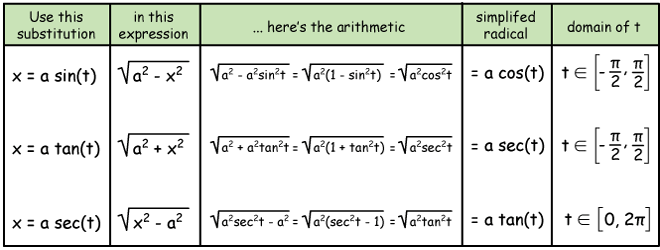PDF

### Trig Sum Identities

The sum identities are the expressions which are used to find out the sum fo two angles of a function. The sum identities obtained can be used to find the angle sum of any particular function.

Here we have given a table depicting the sum identities.PDF

### Simplifying Trig Identities

Simplifying a trigonometric identity is useful for solving trigonometric equations with higher radicals.

Here is a video explaining how you can simplify identities.

### Power Reducing Trig Identities

Power-reducing formulas are used to reduce the power of the radicals in an expression. This can be obtained by using half-angle or double angle identities.

Here we have provided you with the power reducing formulas which can be used to solve expressions with higher radicals.PDF

So we have covered through this article all aspects of trigonometric identities and much more. These identities are very useful for teaching trigonometric concepts to students. And undoubtedly, it is required by astronomers, physicists, architects, to solve many problems and conduct various experiments.

Hence trigonometry forms an important part of the school curriculum and forms the foundation for higher Physics and Mathematics. We hope you have found the information useful and it has helped you understand the concepts of trigonometry.